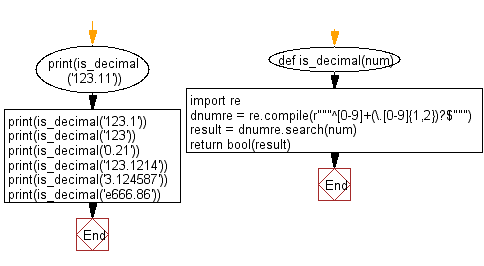﻿ Python: Check a decimal with a precision of 2 - w3resource# Python: Check a decimal with a precision of 2

## Python Regular Expression: Exercise-48 with Solution

Write a Python program to check a decimal with a precision of 2.

Sample Solution:-

Python Code:

``````def is_decimal(num):
import re
dnumre = re.compile(r"""^[0-9]+(\.[0-9]{1,2})?\$""")
result = dnumre.search(num)
return bool(result)

print(is_decimal('123.11'))
print(is_decimal('123.1'))
print(is_decimal('123'))
print(is_decimal('0.21'))

print(is_decimal('123.1214'))
print(is_decimal('3.124587'))
print(is_decimal('e666.86'))
```
```

Sample Output:

```True
True
True
True
False
False
False
```

Pictorial Presentation:Flowchart:Python Code Editor:

Have another way to solve this solution? Contribute your code (and comments) through Disqus.

What is the difficulty level of this exercise?

Test your Python skills with w3resource's quiz

﻿

## Python: Tips of the Day

For-else construct useful when searched for something and find it:

```# For example assume that I need to search through a list and process each item until a flag item is found and
# then stop processing. If the flag item is missing then an exception needs to be raised.

for i in mylist:
if i == theflag:
break
process(i)
else:
raise ValueError("List argument missing terminal flag.")
```